# Area Integral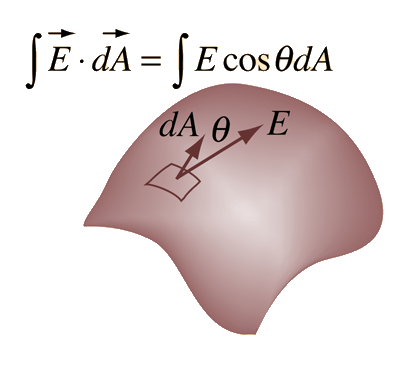An area integral of a vector function E can be defined as the integral on a surface of the scalar product of E with area element dA. The direction of the area element is defined to be perpendicular to the area at that point on the surface.
 The outward directed surface integral over an entire closed surface is denoted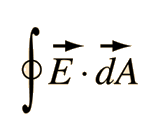It is appropriate for such physical applications as Gauss' law.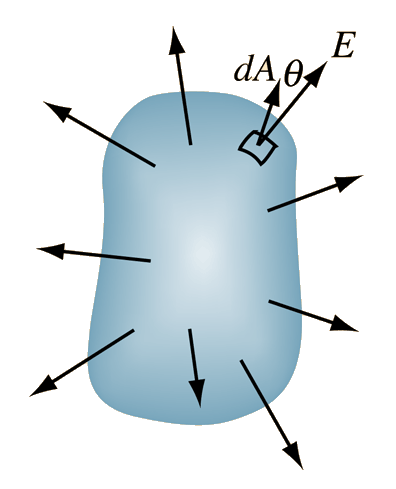Index

 HyperPhysics*****HyperMath*****Calculus R Nave
Go Back

# Line Integral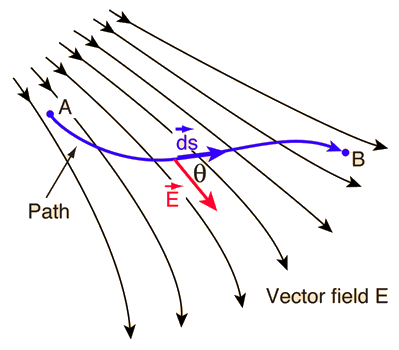Vector functions such as electric field and magnetic field occur in physical applications, and scalar products of these vector functions with another vector such as distance or path length appear with regularity. When such a product is summed over a path length where the magnitudes and directions change, that sum becomes an integral called a line integral.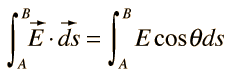A line integral is also used for the general definition of work in mechanics.

### Applications

Index

 HyperPhysics*****HyperMath*****Calculus R Nave
Go Back

# Applications of Line Integrals

The line integral of electric field around a closed loop is equal to the voltage generated in that loop (Faraday's law):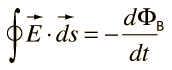Such an integral is also used for the calculation of voltage difference since voltage is work per unit charge. Calculating the voltage difference near a point charge is a good example.

The line integral of a force over a path is equal to the work done by that force on the path.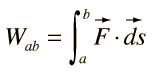Index

 HyperPhysics*****HyperMath*****Calculus R Nave
Go Back